# 论文解读：ACL2021 NER | 基于模板的BART命名实体识别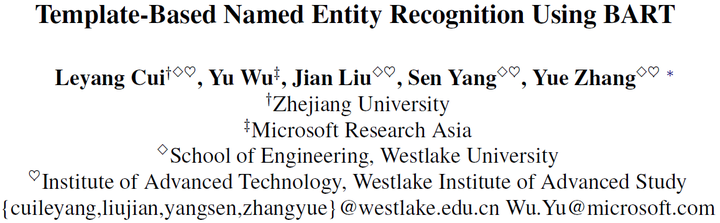## 0、摘要

• 小样本NER：源领域数据多，目标领域数据少
• 现有方法：基于相似性的度量
• 缺点：不能利用模型参数中的知识进行迁移
• 提出基于模板的方法
• NER看作一种语言模型排序问题，seq2seq框架
• 原始句子和模板分别作为源序列和模板序列，由候选实体span填充
• 推理：根据相应的模板分数对每个候选span分类
• 数据集
• CoNLL03 富资源
• MIT Movie、MIT Restaurant、ATIS 低资源

## 1、介绍

• NER：NLP基础任务，识别提及span，并分类
• 神经NER模型：需要大量标注数据，新闻领域很多，但其他领域很少
• 理想情况：富资源 知识迁移到 低资源
• 实际情况：不同领域实体类别不同
• 训练和测试：softmax层和crf层需要一致的标签
• 新领域：输出层必须再调整和训练
• 最近，小样本NER采用距离度量：训练相似性度量函数
• 优：降低了领域适配
• 缺：(1)启发式最近邻搜索，查找最佳超参，未更新网络参数，不能改善跨域实例的神经表示；(2)依赖源域和目标域相似的文本模式
• 提出基于模板的方法
• 利用生成PLM的小样本学习潜力，进行序列标注
• BART由标注实体填充的预定义模板微调
• 实体模板：<candidate_span> is a <entity_type> entity
• 非实体模板：<candidate_span> is not a named entity
• 方法优点：
• 可有效利用标注实例在新领域微调
• 比基于距离的方法更鲁棒，即使源域和目标域在写作风格上有很大的差距
• 可应用任意类别的NER，不改变输出层，可持续学习
• 第一个使用生成PLM解决小样本序列标注问题
• Prompt Learning（提示学习）

## 2、方法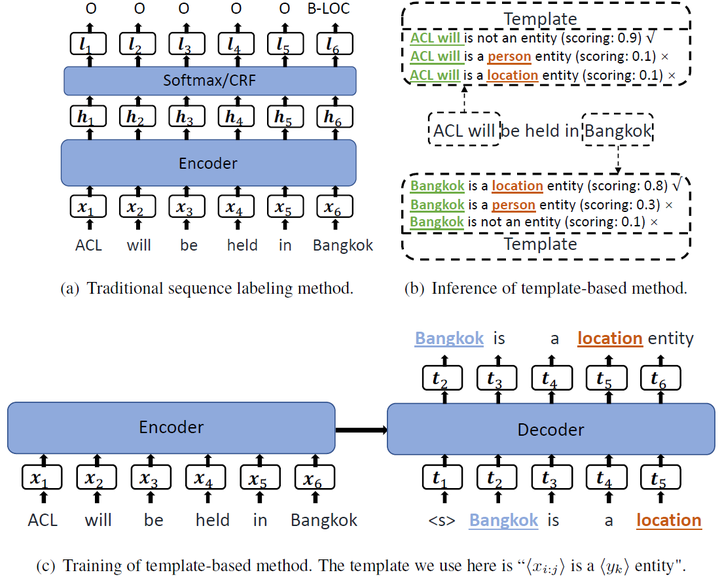### 2.1、创建模板

• 将NER任务看作是seq2seq框架下的LM排序问题
• 标签集 entity_type：\mathbf{L}=\{l_1,...,l_{|L|}\}L={l1​,...,lL∣​}，即{LOC, PER, ORG, …}
• 自然词：\mathbf{Y}=\{y_1,...,y_{|L|}\}Y={y1​,...,yL∣​}，即{location, person, orgazation, …}
• 实体模板：\mathbf{T}^{+}_{y_k}=\text{<candidate\_span> is a location entity.}Tyk​+​=<candidate_span> is a location entity.
• 非实体模板：\mathbf{T}^{-}=\text{<candidate\_span> is not a named entity.}T−=<candidate_span> is not a named entity.
• 模板集合：\mathbf{T}=[\mathbf{T}^{+}_{y_1},...,\mathbf{T}^{+}_{y_{|L|}},\mathbf{T}^{-}]T=[Ty1​+​,...,TyL∣​+​,T−]

### 2.2、推理

• 枚举所有的span，限制n-grams的数量1~8，每个句子有8n个模板
• 模板打分：\mathbf{T}_{{y_k},x_{i:j}}=\{t_1,...,t_m\}Tyk​,xi:j​​={t1​,...,tm​}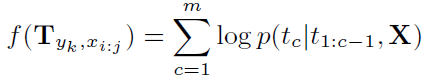• x_{i:j}xi:j​实体得分最高
• 如果存在嵌套实体，选择得分较高的一个

### 2.3、训练

• 金标实体用于创建模板
• 实体x_{i:j}xi:j​的类型为y_kyk​，其模板为：\mathbf{T}^{+}_{y_k,x_{i:j}}Tyk​,xi:j​+​
• 非实体x_{i:j}xi:j​，其模板为：\mathbf{T}^{-}_{x_{i:j}}Txi:j​−​
• 构建训练集：
• 正例：(\mathbf{X}, \mathbf{T}^+)(X,T+)
• 负例：(\mathbf{X}, \mathbf{T}^-)(X,T−)，随机采样，数量是正例的1.5倍
• 编码：\mathbf{h}^{enc}=\text{ENCODER}(x_{1:n})henc=ENCODER(x1:n​)
• 解码：\mathbf{h}_c^{dec}=\text{DECODER}(h^{enc}, t_{1:c-1})hcdec​=DECODER(henc,t1:c−1​)
• 词t_ctc​的条件概率：p(t_c|t_{1:c-1},\mathbf{X})=\text{SOFTMAX}(\mathbf{h}_c^{dec}\mathbf{W}_{lm}+\mathbf{b}_{lm})p(tc​∣t1:c−1​,X)=SOFTMAX(hcdec​Wlm​+blm​)
• \mathbf{W}_{lm} \in \mathbb{R}^{d_h\times |V|}Wlm​∈Rdh​×∣V
• 交叉熵loss

## 3、结果

• 不同模板类型的测试结果
• 选择前三个模板，分别训练三个模型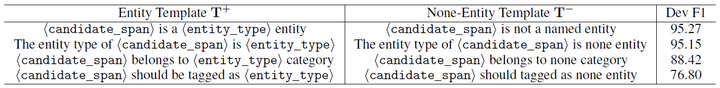• 实验结果
• 最后一行是三模型融合，实体级投票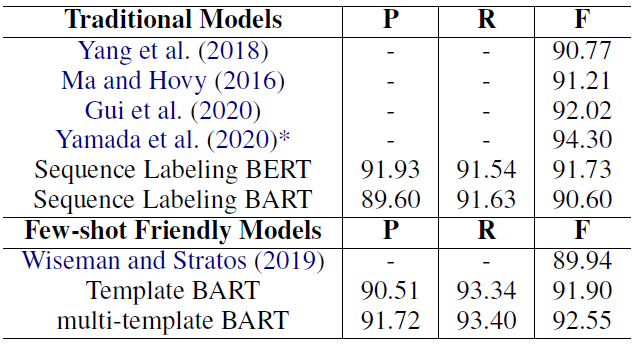posted @ 2021-10-22 11:20  华为云开发者社区  阅读(6)  评论(0编辑  收藏  举报